# 给Java开发者的Scala教程

+关注继续查看

author：Michel Schinz，Philipp Haller

## 2. 第一个例子

object HelloWorld { def main(args: Array[String]): Unit = { println("Hello, world!") }

### 2.1 编译这个例子

>scalac HelloWorld.scala

### 2.2 运行这个例子

>scala -classpath . HelloWorld

## 3 和Java互操作

import java.util.{Date, Locale} import java.text.DateFormat import java.text.DateFormat._ /** * Created by home on 3/11/15. */ object FrenchDate { def main(args: Array[String]): Unit = { val now = new Date val df = getDateInstance(LONG, Locale.FRANCE) println(df format now) }

Scala的import语句和java的几乎一样，不过，更加灵活。一个包得多个类可以在一个import语句里用大括号 括起来一次引用进来。另一个不同是，使用下划线而不是星号引入包中的全部类。所以，第三行import语句引入了 DateFormat的全部成员。

## 4 什么都是对象

Scala是一个纯的面向对象的语言，所以其内部的全部都是对象。包括数字和方法。在这一帮面，Scala和java有 很大的不同。因为，java区分值类型和引用类型，也不允许把方法当做值来处理。

### 4.1 数字和对象

1 + 2 * 3 / x

(1).(((2).*(3))./(x))

### 4.2 方法也是对象

object Timer { def oncePerSecond(callback: () => Unit): Unit = { while (true) { callback(); Thread sleep 1000 def timeFlies(): Unit = { println("time flies like an arrow...") def main(args: Array[String]): Unit = { oncePerSecond(timeFlies) }

### 4.2.1 匿名方法

object TimerAnonymous { def oncePerSecond(callback: () => Unit): Unit = { while (true) { callback(); Thread sleep 1000 def main(args: Array[String]): Unit = { oncePerSecond(() => println("time flies like an arrow...")) }

## 5 类

class Comlex(real: Double, imaginary: Double) { def re() = real def im() = imaginary }

### 5.1 无参数方法

def main(args: Array[String]): Unit = { val c = new Complex(1.3, 4.6) println("Imaginary part: " + c.im()) }

class Complex(real: Double, imaginary: Double) { def re = real def im = imaginary object TimerAnonymous { def main(args: Array[String]): Unit = { val c = new Complex(1.3, 4.6) println("Imaginary part: " + c.im) }

### 5.2 继承和override

Scala的类全部都继承自一个超类。如果没有显示的给出超类，如上面的Complex类，那么则默认的继承自scala.AnyRef。 在Scala中也可以override超类的方法。在override的时候需要用override关键字明确的标明。如：

class Complex(real: Double, imaginary: Double) { def re = real def im = imaginary override def toString() = "" + re + (if (im < 0) "" else "+") +im + "i" }

## 6 case类和模式匹配

abstract class Tree case class Sum(l: Tree, r: Tree) extends Tree case class Var(n: String) extends Tree case class Const(v: Int) extends Tree

• new关键字在创建实例的时候不是必须的（如：Const(5)，不用写new Const(5)),
• getter方法自动根据构造函数的参数（如Const类的实例c，从c中取构造函数参数v的值可以用c.v来取得),
• 提供默认的toString方法，并按照最基本的调用方法打印。如：x+1打印为：Sum(Var(x), Const(1)),
• 这些类的实例可以用模式匹配来分解，这个下面会讲到。

{case "x" => 5}

type Environment = String => Int

def eval(t: Tree, env: Environment): Int = t match { case Sum(l, r) => eval(l, env) + eval(r, env) case Var(n) => env(n) case Const(v) => v }

1. 检查表达式是不是Sum，如果是则其包含了左子树和右子树，分别为lr。之后继续推导箭头后面的 表达式；
2. 如果第一个表达是没有成功执行，也许这个树不是一个Sum。继续检查如果t（形参）是否为Var，如果是则将Var节点 包含的值和n变量绑定并继续处理右手表达式。
3. 如果第二个检查也失败了，那么t既不是Sum也不是一个Var。检查t是否为一个Const，如果是则将Const节点包含的 值和v变量绑定并继续处理右手表达式。
4. 最后，如果全部检查失败，则抛出一个异常。在这里，只会在更多的Tree子类被定义的时候发生。

• 当使用方法时，添加新的节点更加容易：只需要定义一个新的Tree子类。另一方面来说：给树添加一种新的操作 （比如加法之外的减、乘除等）就比较麻烦了。因为，需要给每一个子类都做出相应的修改。
• 使用模式匹配的时候，情况正好相反。添加一个新的节点需要修改全部的模式匹配方法。添加一个操作就很简单了 ，只需要定义另外的一个方法。

## 7 接口（Traits）

trait Ord { def <(that: Any): Boolean def <=(that: Any): Boolean = (this < that) || (this == that) def >(that: Any): Boolean = !(this <= that) def >=(that: Any): Boolean = !(this < that) }

class Date(y: Int, m: Int, d: Int) extends Ord { def year = y def month = m def day = d override def toString(): String = year + "-" + month + "-" + day }

override def equals(that: Any): Boolean = that.isInstanceOf[Date] && { val o = that.asInstanceOf[Date] o.day == day && o.month == month && o.year == year }

def <(that: Any): Boolean = { if (!that.isInstanceOf[Date]) sys.error("cannot compare " + that + " and a Date") val o = that.asInstanceOf[Date] (year < o.year) || (year == o.year && (month < o.month || (month == o.month && day < o.day))) }

## 8 泛型

class Reference[T] { private var contents: T = _ def set(value: T) { contents = value def get: T = contents }

class IntegerReference { def main(args: Array[String]): Unit = { val cell = new Reference[Int] cell.set(13) println("Reference contains the half of " + (cell.get * 2)) }

get之后直接计算，无需再做任何的类型转换。

## 9 结语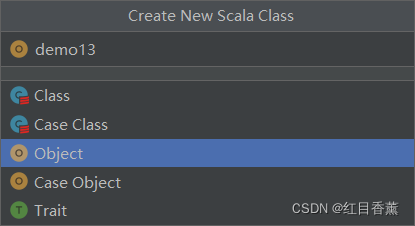27 0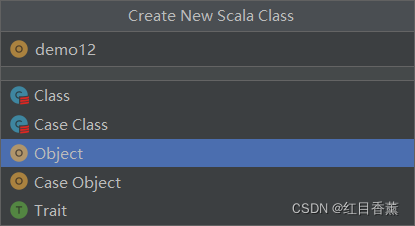68 0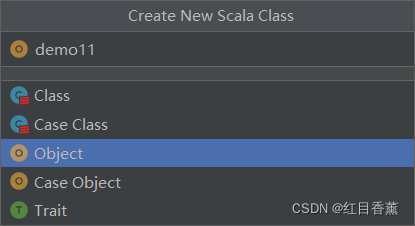41 0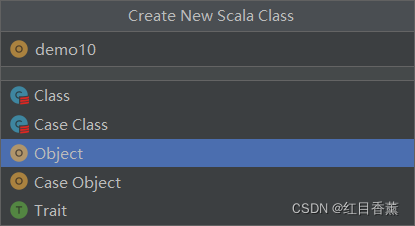38 0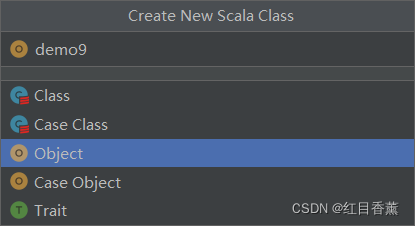40 0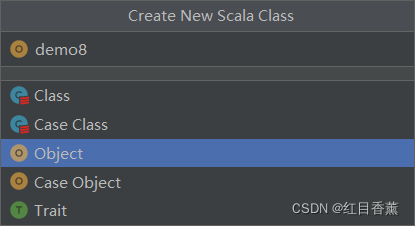43 0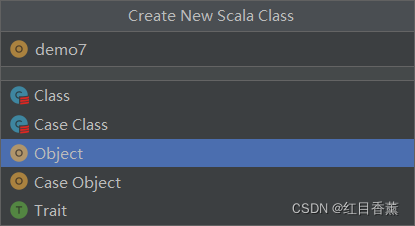33 0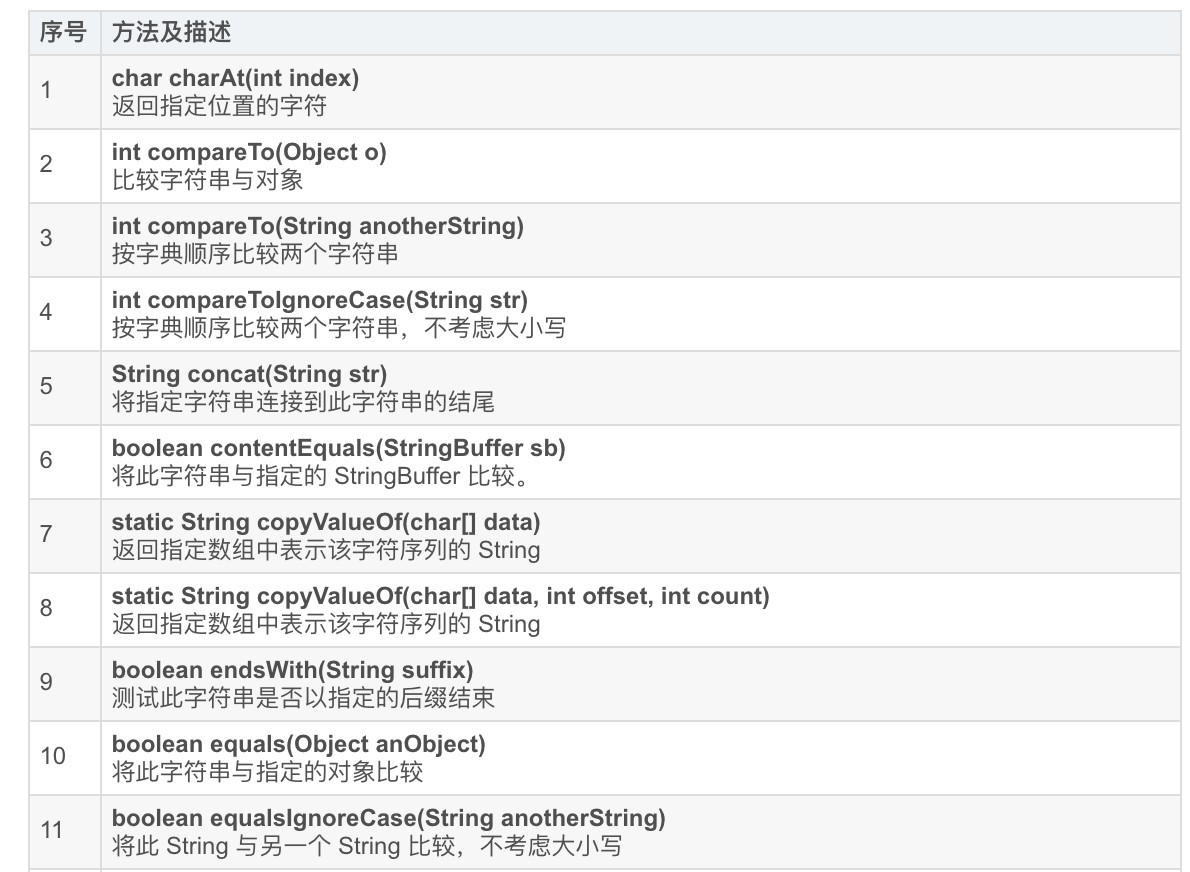22 0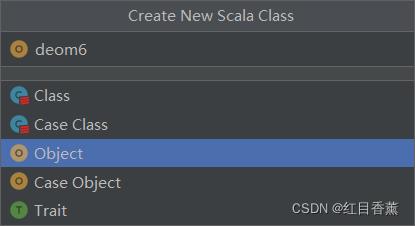33 0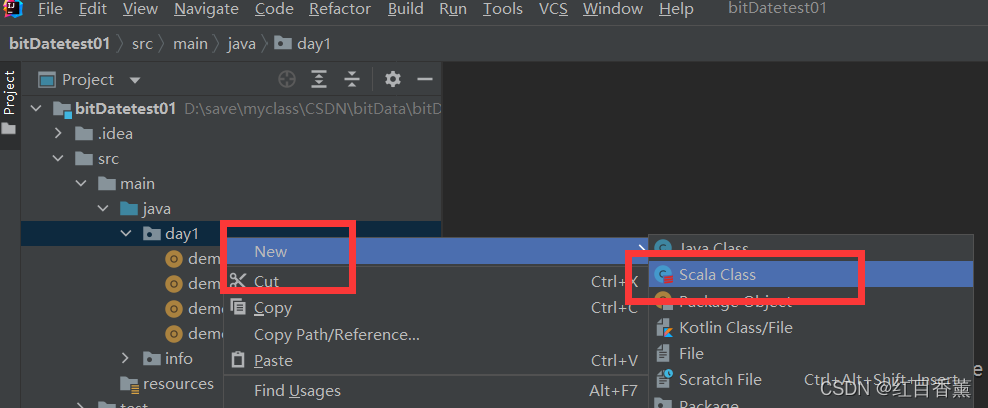18 0

Scala完全自学手册图文教程65423

Java Web开发系列课程 - Spring框架入门2977325

Java Web开发-Web应用、Tomcat、HTTP请求与响应137217

Scala核心编程 - 基础726147

Scala核心编程 - 进阶333142

Java Spring Boot 2.6.0开发实战-1024程序员节创造营公益课9335

Just Enough Scala for Spark

JDK8新特性与生产-for“华东地区scala爱好者聚会”

JDK8新特性与生产-for“华东地区scala爱好者聚会”